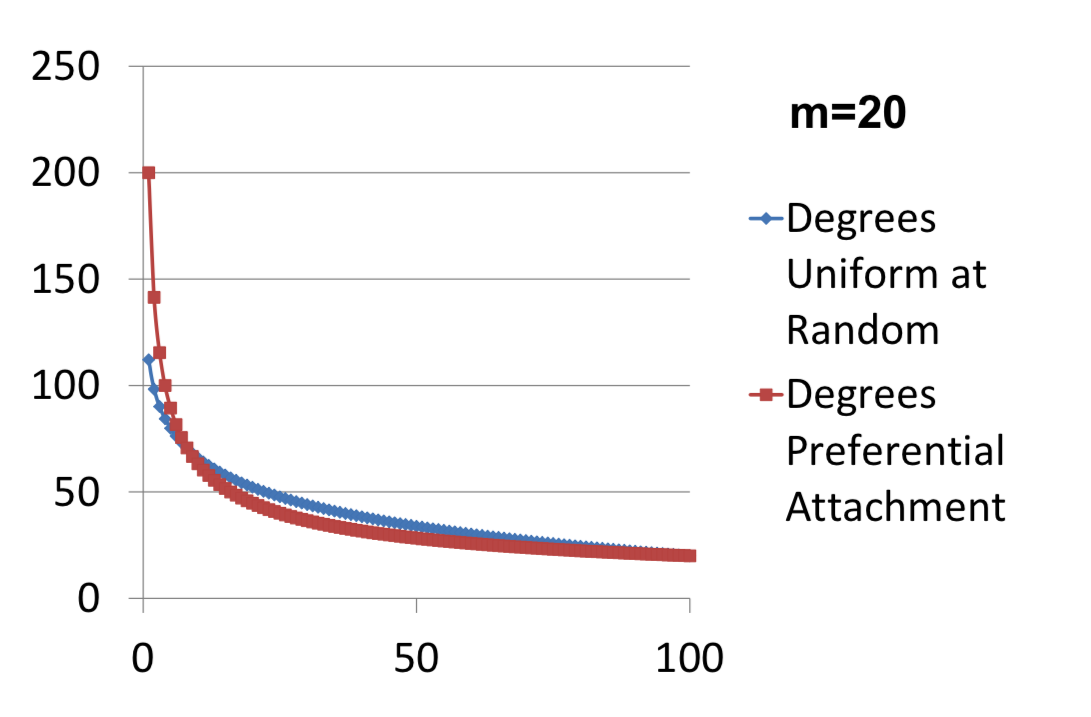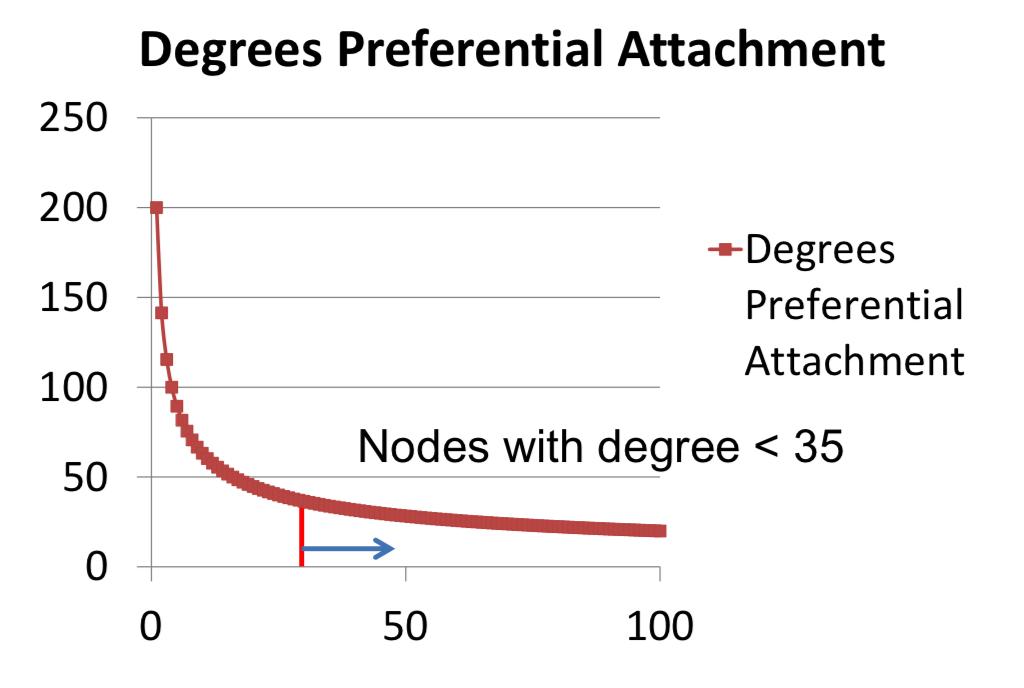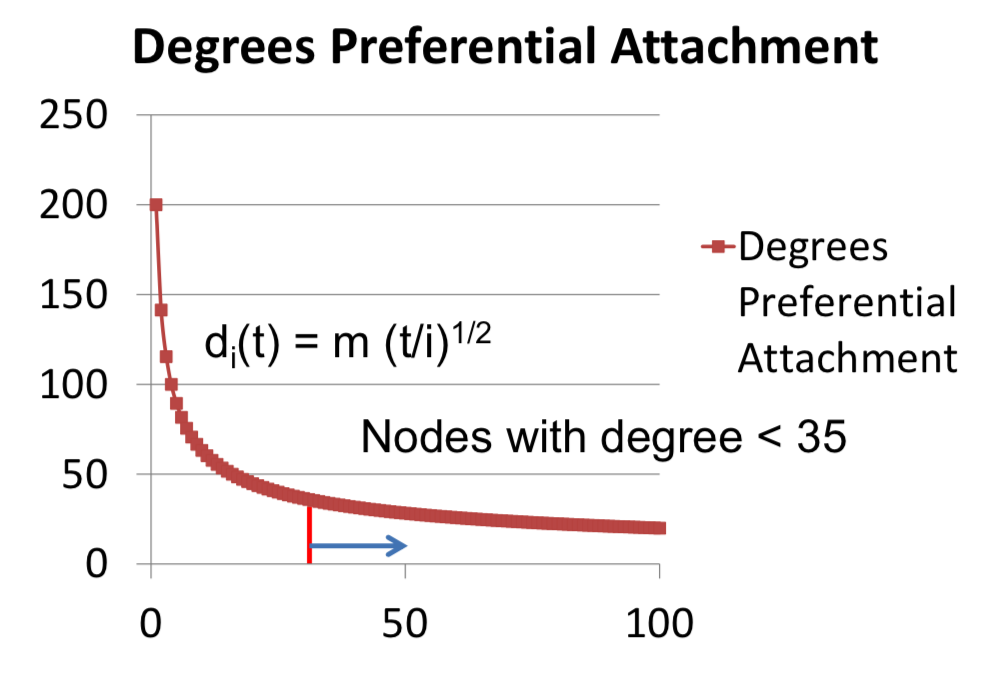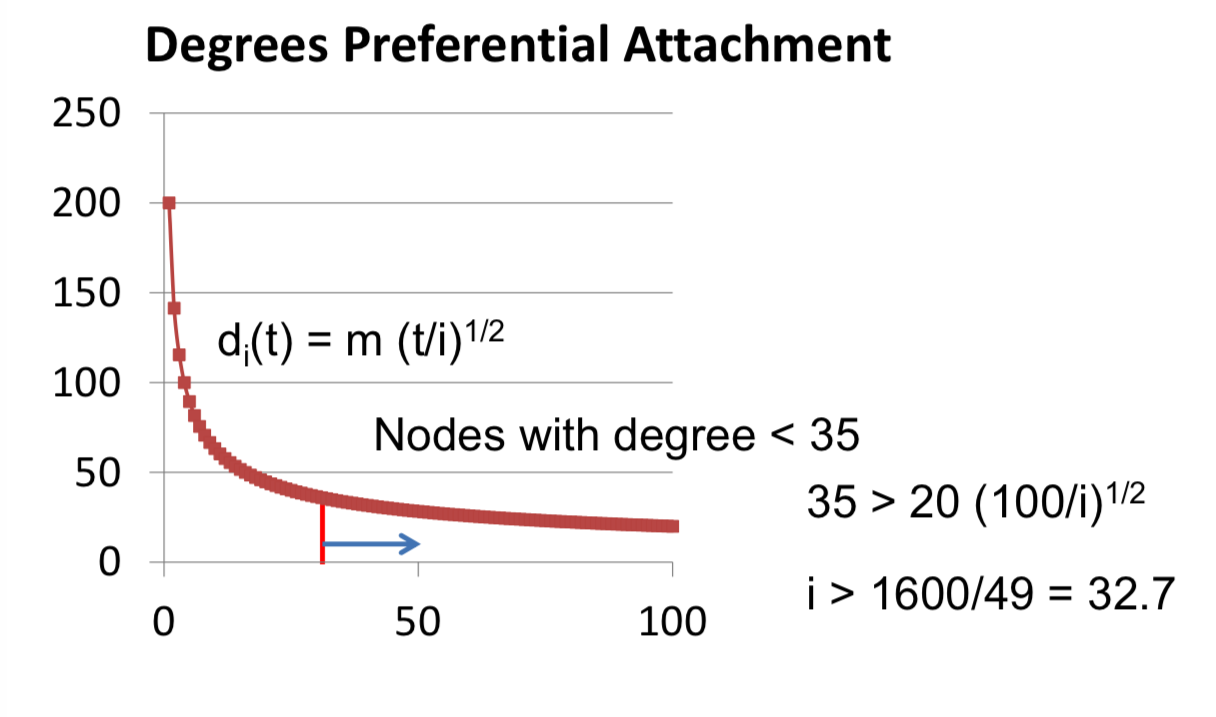# 3.3 Preferential Attachment¶

• Newborn nodes form m links to existing nodes

• born one each period, so time just keeps track of birth dates. The important thing is the formation process is going to be different.
• tm links in total

• So at some time t, the number of links that are going to be in the system are going to tm links in total. t nodes born it reached form m links so tm in total.
• total degree is 2tm

• So each link has two different nodes that are going to get to count it as a link, so we have 2tm as a total number of degrees in the system at any point in time.
• Probability of attaching to i is $d_i(t)/2tm$
• And the probability of attaching to a given node is proportional to it's degree compared to the overall degree in the society. So nodes that have very high degree are going to have higher chances, and so in particular, the probability of attaching to node i, is going to be it's degree divided by 2tm($d_i(t)/2tm$), which is very different than the $m/t$ that we had before. So before, every node that was out there had an equal chance of getting a new link. Now the chance of getting a new link is proportional to your degree, compared to the overall degree in the society at that time period.

## Distribution of Expected Degrees¶

• When you're born, you form m links($d_i(i) = m$, so that's the initial starting condition. How does your degree change? Well, you're getting new links at a rate which is proportional to how your degree compares to 2t over m($d_i(t)/2tm$), there's m new links being formed per unit of time. Therefore this differential has this equation:

• $dd_i(t) / dt = md_i(t) / 2tm = d_i(t) / 2t$ and $d_i(i) = m$
• $d_i(t) = m(t/i)^{1/2}$ (equation for degree: the degree looks like m times the time over the birth date raised to the one half power)
• Expected degree for node i born at m<i<t is

• $d_i(t) = m(t/i)^{1/2}$
• Nodes that have expected degree less than d at some time t are those such that $m(t/i)^{1/2} < d$
• $i > tm^2/d^2$
• $F_t(d) = (t - tm^2/d^2)/t = 1 - (m/d)^2$
• $f_t(d) = 2m^2/d^3$ (the derivative of $F_t(d)$ and look at what's the density function for the distribution generated by preferential attachment. Then $2m^2/d^3 = (2m^2)d^{-3}$, so we have a power law)## Power Law¶

• $log(f(d)) = log(2m^2) - 3log(d)$
• Why 3 in 3log(d)? Well, that came from the fact that the change in degree, over time had a 2 in the denominator($dd_i(t) / dt = d_i(t) / 2t$), and then when we do integration and so forth we came out with a 3, but basically that's coming from the fact that there's a certain number of links present in this society. And if you want to vary this, you can actually produce different variations on this coefficient. And the way that you can produce different variations on this coefficient is just having the number of links being formed at any point in time, either grow or shrink over time, so you can have the population, the number of newborn nodes not be a constant one period but changing over time, and that's allow you to control this variable here. So a slightly richer variation of Preferential Attachment can adjust that.
• The important thing is that the older nodes are gaining, things that are richer, faster and faster time meant that we end up with these fat tails and power law.